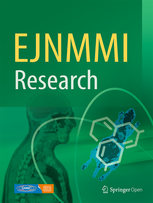New publication: Optimized dual-time-window protocols for quantitative [18F]flutemetamol and [18F]florbetaben PET studies

Background

A long dynamic scanning protocol may be required to accurately measure longitudinal changes in amyloid load. However, such a protocol results in a lower patient comfort and scanning efficiency compared to static scans. A compromise can be achieved by implementing dual-time-window protocols. This study aimed to optimize these protocols for quantitative [18F]flutemetamol and [18F]florbetaben studies.

Methods

Rate constants for subjects across the Alzheimer’s disease spectrum (i.e., non-displaceable binding potential (BPND) in the range 0.02–0.77 and 0.02–1.04 for [18F]flutemetamol and [18F]florbetaben, respectively) were established based on clinical [18F]flutemetamol (N = 6) and [18F]florbetaben (N = 20) data, and used to simulate tissue time-activity curves (TACs) of 110 min using a reference tissue and plasma input model. Next, noise was added (N = 50) and data points corresponding to different intervals were removed from the TACs, ranging from 0 (i.e., 90–90 = full-kinetic curve) to 80 (i.e., 10–90) minutes, creating a dual-time-window. Resulting TACs were fitted using the simplified reference tissue method (SRTM) to estimate the BPND, outliers (≥ 1.5 × BPND max) were removed and the bias was assessed using the distribution volume ratio (DVR = BPND + 1). To this end, acceptability curves, which display the fraction of data below a certain bias threshold, were generated and the area under those curves were calculated.

Results

[18F]Flutemetamol and [18F]florbetaben data demonstrated an increased bias in amyloid estimate for larger intervals and higher noise levels. An acceptable bias (≤ 3.1%) in DVR could be obtained with all except the 10–90 and 20–90-min intervals. Furthermore, a reduced fraction of acceptable data and most outliers were present for these two largest intervals (maximum percentage outliers 48 and 32 for [18F]flutemetamol and [18F]florbetaben, respectively).

Conclusions

The length of the interval inversely correlates with the accuracy of the BPND estimates. Consequently, a dual-time-window protocol of 0–30 and 90–110 min (=maximum of 60 min interval) allows for accurate estimation of BPND values for both tracers.

The article has been published open access and can be read here: https://doi.org/10.1186/s13550-019-0499-4More News